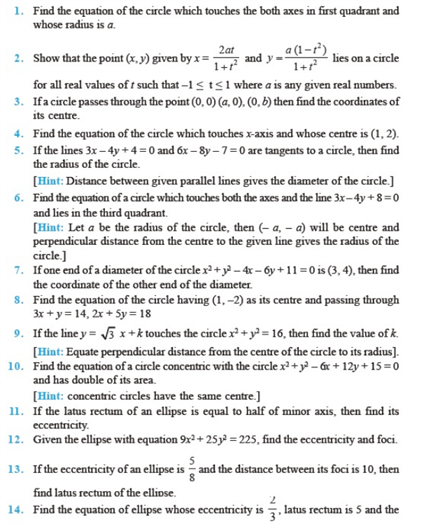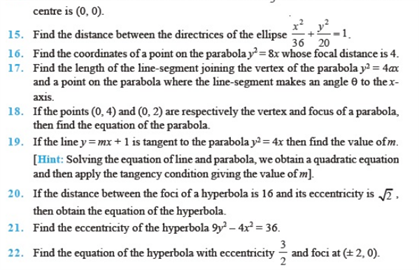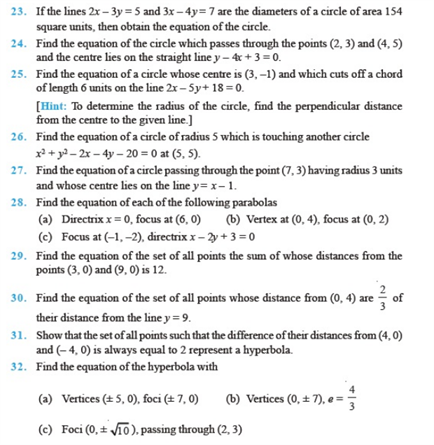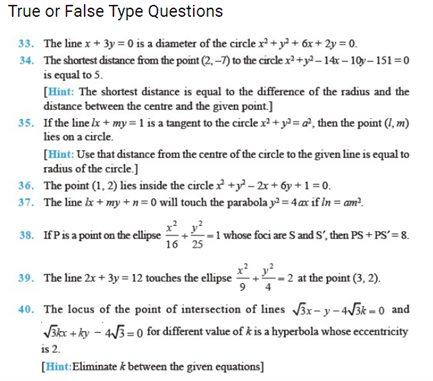# Chapter 11 - Conic Sections

Some of the most important Conic Sections Class 11 questions i.e chapter 11 are given here. These chapter 11 class 11 maths questions cover several short answer type questions, long answer type questions and HOTS questions that are crucial for CBSE class 11 exams. Here, some of the important Conic Sections questions class 11 from NCERT are also included.

Also, Check:

## Conic Sections Important Questions For Class 11 (Chapter 11)

These class 11 Conic Sections questions are categorized into short answer type questions and long answer type questions. These questions cover various concepts which will help class 11 students to develop problem-solving skills for the exam.

1) Find the equation of a circle which passes through the origin and cuts off intercepts -2 and 3 from the axis and the y-axis respectively. (Solution: x2 + y2 + 2x -3y)

2) Find the equation of the circle passing through the points – (0,0)(5,0) and (3,3). (Solution: x2 + y2 – 5x -y =0), centre (5/2 , ½) and radius =  √ 26/2).

3) If the distance between the foci of a hyperbola is 16 and eccentricity is  √ 2, then obtain its equation. (Solution: x2 – y2 =32)

4) If a latus rectum of an ellipse subtends a right angle at the centre of the ellipse, then write the eccentricity of the ellipse. (Solution:  (√ 5 – 1) / 2)

5) Find the equation of the ellipse whose foci are (4,0) and (-4,0), eccentricity = ⅓. (Solution: x2 / 9 + y2/8 = 16)

6) Write the equation of the parabola whose vertex is at (-3,0) and the directrix is (x + 5 ) = 0. (Solution: y2 = 8(x + 3))

7) AB is a double ordinate of a parabola y2 = 4px. Find the locus of its points of trisection. (Solution: 9y2 =4px)

8) Find the equation of the parabola whose focus is (1, -1) and whose vertex is (2,1). Also, find its axis and latus- rectum). (Solution: 4 √ 5).

9) Find the equation of the circle which circumscribes the triangle formed by the lines x = 0, y = 0 and lx +my = 1. (Solution: x2 + y2 – (1/l)x – (1/m)y = 0)

10) Prove that the points  (9,1) ( 7,9) (-2, 12) and (6,10) are concyclic.

### NCERT Questions Class 11 Chapter 11